Homework Help Question & Answers

# g. Find the support forces A, at A and D, at D. A, at A D,...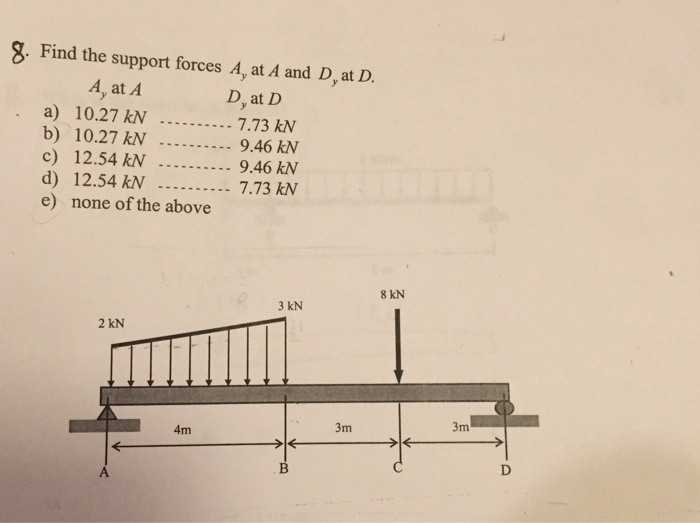g. Find the support forces A, at A and D, at D. A, at A D, at D a) 10.27 kN.. 7.73 kN b) 10.27 kN 9.46 kN c) 12.54 kN 9.46 kN d) 12.54 kN e) none of the above 7.73 kN 8 kN 3 kN 2 kN 3m 4m

#### Homework Answers

Answer #1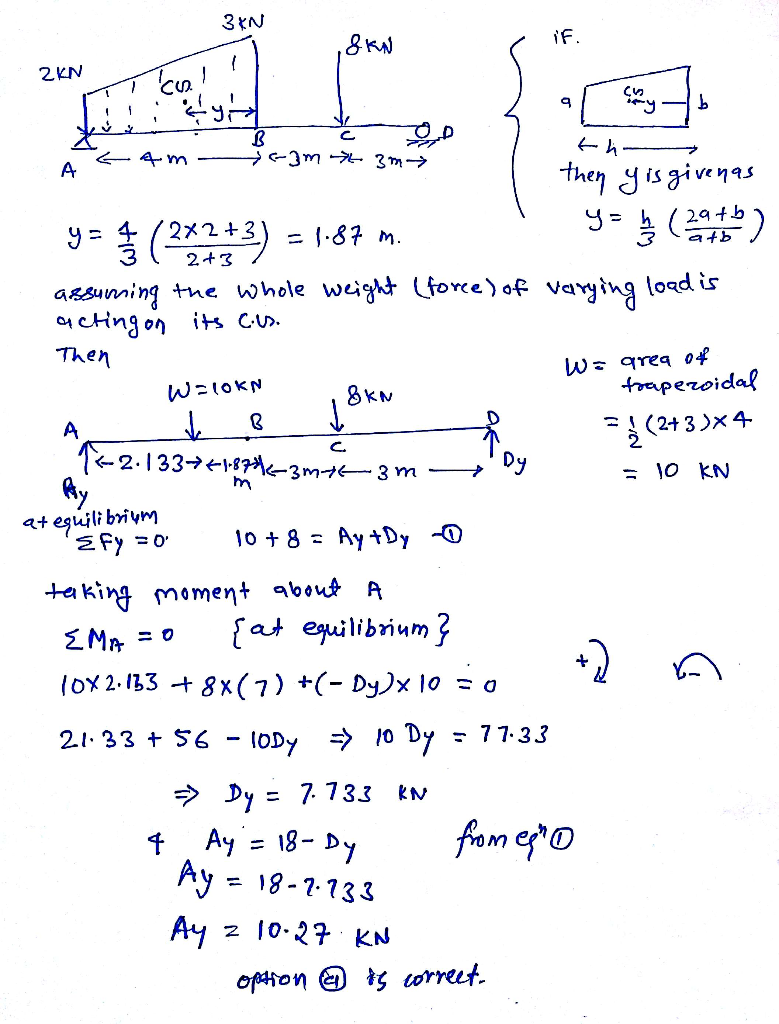Know the answer?
Your Answer:

#### Post as a guest

Your Name:

What's your source?

#### Earn Coin

Coins can be redeemed for fabulous gifts.

Not the answer you're looking for? Ask your own homework help question. Our experts will answer your question WITHIN MINUTES for Free.
Similar Homework Help Questions
• ### The following truss is subjected to vertical loads of 20 KN at joints E and D....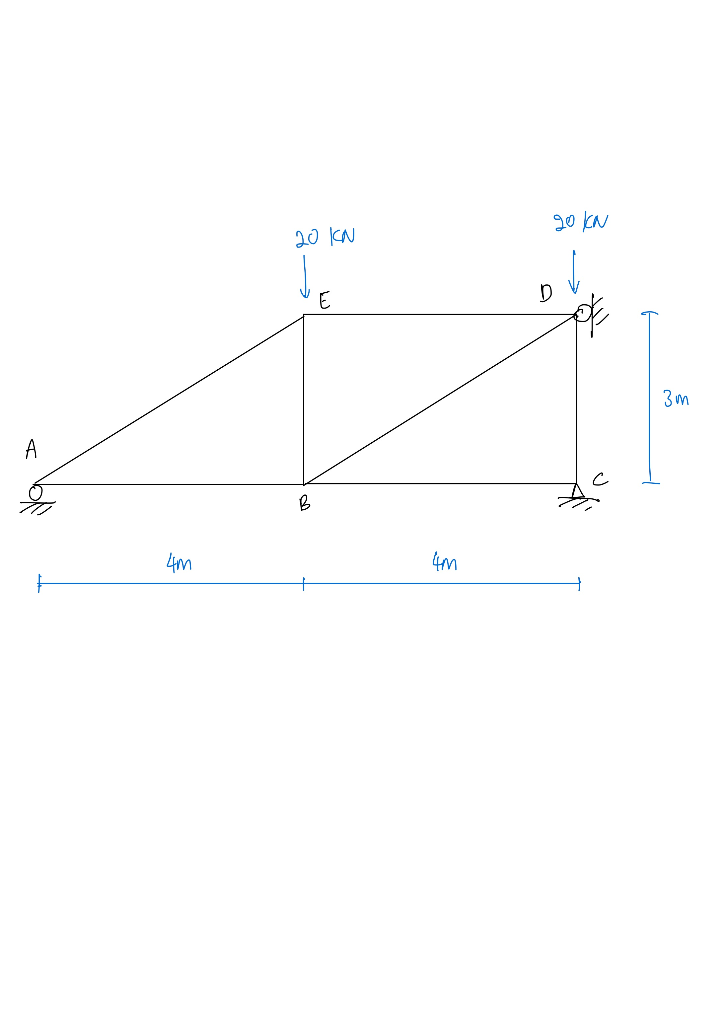The following truss is subjected to vertical loads of 20 KN at joints E and D. In addition to the loads, support A settles by 5 mm and member AB and BC are subjected to a temperature drop of 50°C. Given Young’s modulus, E = 200 GPa, cross sectional area for each member, A = 500 mm2 and coefficient of thermal expansion of, α = 1.25 x 10-5/°C. Find the internal forces in each member using force method. 20 KN...

• ### Please draw a fbd too. Q7. Determine the support reactions at B and C. 2 kN...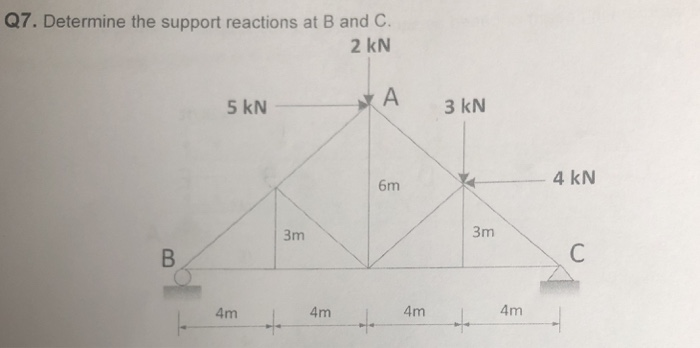Please draw a fbd too. Q7. Determine the support reactions at B and C. 2 kN 5 kN A 3 kN 4 kN 6m 3m 3m 4m4m4m 4m

• ### Find the internal forces for the truss shown below in member BF D 4 M EL...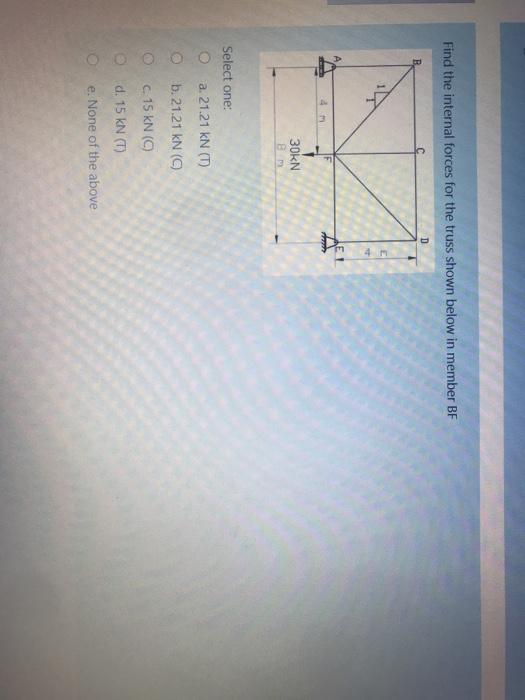Find the internal forces for the truss shown below in member BF D 4 M EL F 4 m 30KN - Select one: O a. 21.21 kN (T) b.21.21 KN (C) c. 15 kN (0) d. 15 kN (T) e. None of the above

• ### find the distribution factor of the beam between Hinge and Fix-End Support A. 2/3 B. 3/5...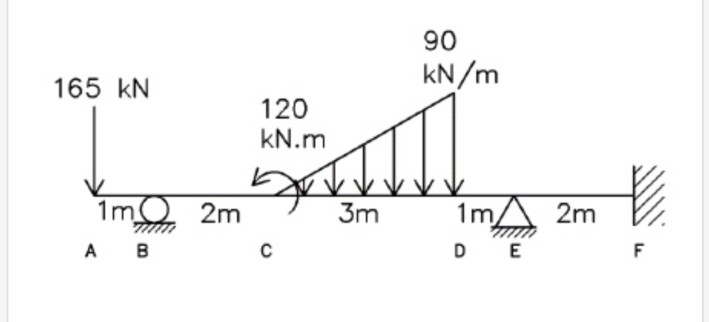find the distribution factor of the beam between Hinge and Fix-End Support A. 2/3 B. 3/5 C. 1/2 D. 3/8 90 kN/m 165 KN 120 KN.m 3m 1m 2m А в 1mA 2m с D E F

• ### Use force method. Find all the member forces for the truss shown. 120 kN 360 kN...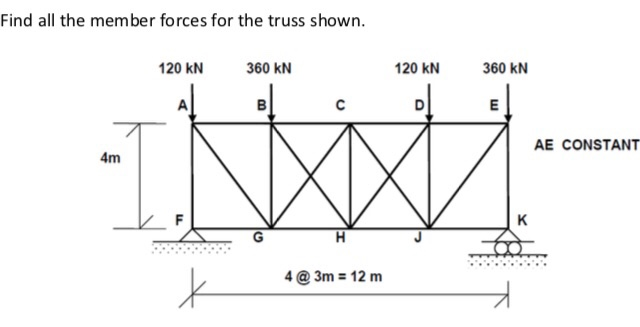Use force method. Find all the member forces for the truss shown. 120 kN 360 kN 120 kN 360 kN AE CONSTANT 4m [email protected]=12m

• ### 4) (a) Determine the forces in bar BC, IC, IC, BC, CI, JC, and D'D of...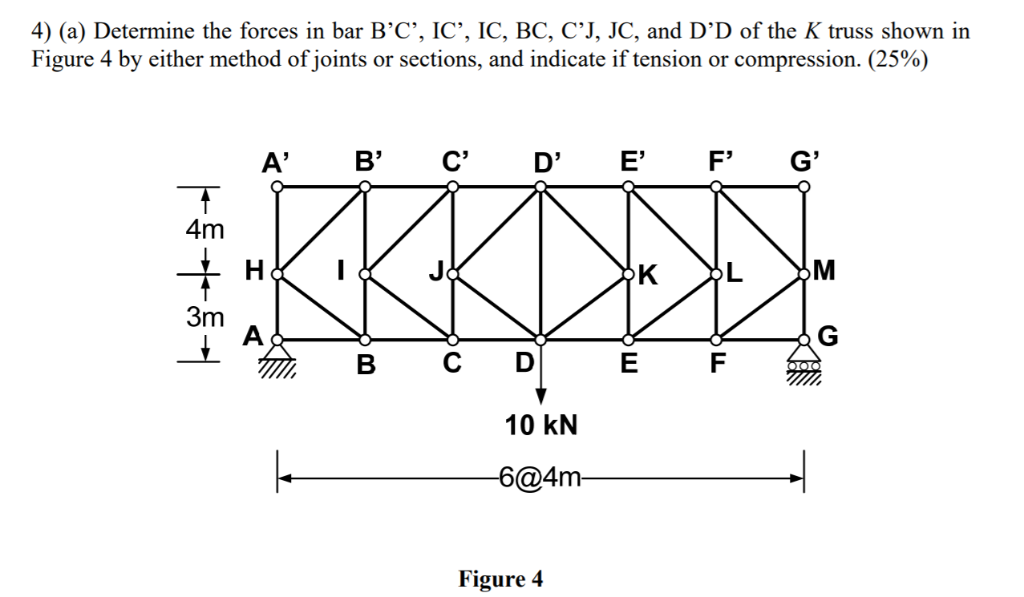4) (a) Determine the forces in bar BC, IC, IC, BC, CI, JC, and D'D of the K truss shown in Figure 4 by either method ofjoints or sections, and indicate if tension or compression. (25%) A' B' CDE' F'G' 4m 3m B C D E F 10 kN [email protected] Figure 4

• ### A) Find the reaction forces a fixed support (point a), the pin at point c and...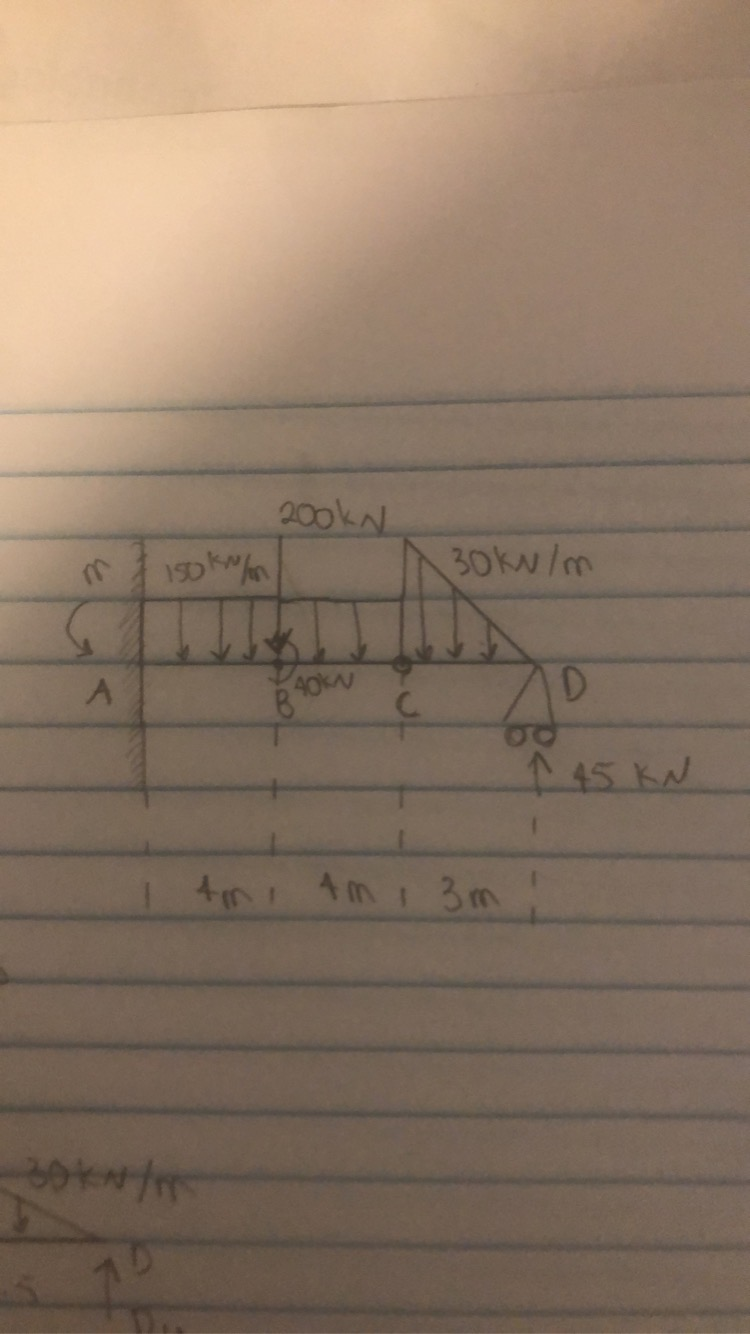A) Find the reaction forces a fixed support (point a), the pin at point c and the roller at point d. B) Draw the stress and bending moment diagrams. 200kn iskin m 30kw/m 1 45KN Ami Am, 3m

• ### Q1 a) List all the zero force members in the truss below Find Forces in AB,...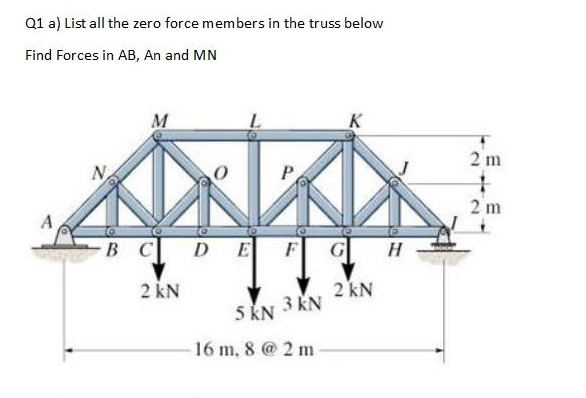Q1 a) List all the zero force members in the truss below Find Forces in AB, An and MN 2 m B C D E F G H I 2 KN 2 KN 5 KN 3 KN - 16 m, 8 @ 2 m

• ### please short summarized answer 5minutes How many zero-force members are there in the truss shown? JB...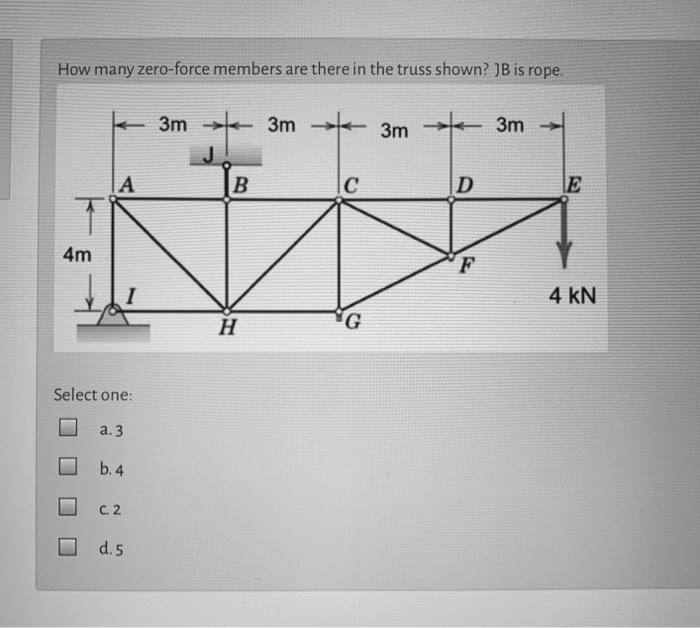please short summarized answer 5minutes How many zero-force members are there in the truss shown? JB is rope. 3m 3m + Зm 3m А B с D 4m - 4 kN H G Select one: a. 3 b. 4 C. 2 d.5 How many zero-force members are there in the truss shown? M L K T N o P 2 m + A 2 m o O O o в с D E F G н 2 kN 2 kN...

• ### 1. (a) Find all the zero-force members of the truss. (b) Determine the reactions at the...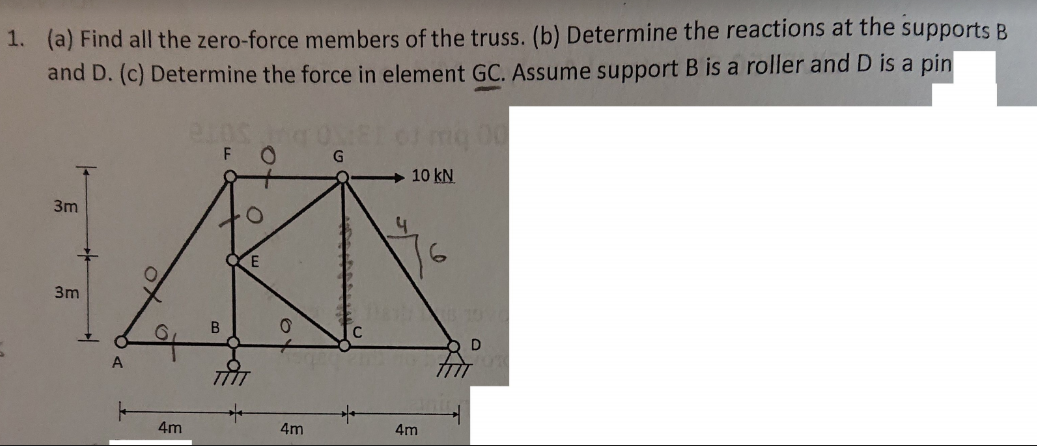1. (a) Find all the zero-force members of the truss. (b) Determine the reactions at the supports B and D. (c) Determine the force in element GC. Assume support B is a roller and D is a pin 10 kN 3m B & 4m

Free Homework App

Scan Your Homework
to Get Instant Free Answers
Need Online Homework Help?

Get Answers For Free
Most questions answered within 3 hours.